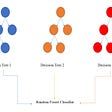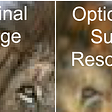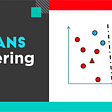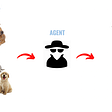# Introduction

K Nearest Neighbors is a lazy learner. It does not build a model as such. Scaling of features is necessary before calculating distances when the features have different ranges.

1. No training required.
2. Very easy to interpret.

1. Need to find the optimum K(no. of neighbors). This might change as new data is added.
2. Problems arise when data is very large or the no. of dimensions is large. This can be solved by using kd-tree and ball tree algorithms.
3. It is sensitive to noisy data.

# Model building

Important points to note:

1. We need to normalize/standardize the numerical features and encode the categorical features to dummies.
2. If the predictor data is a mix of numeric and categorical features, we can only do MinMax scaling of the numerical data. This is to keep the values bounded within [0,1] in the same range as categorical encoded variables.
3. If the predictors are all numeric, we can use Euclidean, Manhattan, Minowski or Mahalanobis distance.
4. If the predictors are only categorical, we can use hamming distance.
5. If the predictors are a mix of of numeric and categorical, we have to choose the distance metric very carefully.
6. When the data has very high dimensions, we suffer from the curse of dimensionality. In this case we restrict to using lower powers in the minkowski distance. We can also resort to dimension reduction techniques and feature selection.
7. The best value of K can be found out by plotting the error and the K.

# Prediction

The new observation is placed in the feature space of the training data.

## Classification

The output class is the modal class for n no. of neighbors.

## Regression

The output estimate is the mean value of n no. of neighbors.

You should keep the no. of neighbors as odd when the no. of classes is even and vice versa to avoid confusion.

# Hyperparameter tuning

• n_neighbors: To set the no. of neighbors
• weights: uniform; distance:
uniform: uniform weight of all points
distance: nearer points have more weight inversely proportional to their distance
• algorithm: the type of algorithm construction.
kd-tree: K dimensional tree will be formed (axis parallel hyperplanes)
ball-tree: the space will be divided into concentric spheres
brute-force: all the distances will be calculated
• leaf_size: the no. of points in the kd-tree or ball tree leaf
• p: Power parameter for the Minkowski metric
• metric: distance metric for the algorithm
• metric_params: additional metric parameter; like covariance matrix for Mahalanobis distance

******************************************************************Example code:

from sklearn.neighbors import KNeighborsRegressor as K{or KNeighborsClassifier}

knn =K(n_neighbors=5)

knn.fit(X_train, y_train)

y_hat = knn.predict(X_test)

******************************************************************

# References

--

--

--

## More from Geek Culture

A new tech publication by Start it up (https://medium.com/swlh).

## Activation maps for deep learning models in a few lines of code## Attention: Fourier Transforms. A giant leap in transformer efficiency.## Adaptive Boosting or Ada Boosting algorithm — Hidden Success behind the many Kaggle Competition## A tutorial on digit recognition with Random Forest Classifier## Recurrent Neural Networks: Part 5## AI super resolution using an optical computer## K-Means Clustering## Convolutional Neural Networks: Part 9## Anupam Misra

Trying to solve the riddle called life.

## Ensembling in Machine Learning.## Unsupervised Learning: Explained, Briefly## Building a QABot using Deep Learning## How to Master TensorFlow Variables in 6 Easy Steps